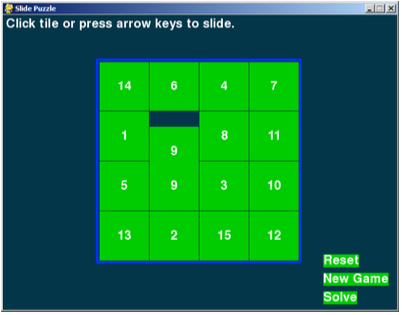# 5.28: The copy() Surface Method

$$\newcommand{\vecs}{\overset { \rightharpoonup} {\mathbf{#1}} }$$ $$\newcommand{\vecd}{\overset{-\!-\!\rightharpoonup}{\vphantom{a}\smash {#1}}}$$$$\newcommand{\id}{\mathrm{id}}$$ $$\newcommand{\Span}{\mathrm{span}}$$ $$\newcommand{\kernel}{\mathrm{null}\,}$$ $$\newcommand{\range}{\mathrm{range}\,}$$ $$\newcommand{\RealPart}{\mathrm{Re}}$$ $$\newcommand{\ImaginaryPart}{\mathrm{Im}}$$ $$\newcommand{\Argument}{\mathrm{Arg}}$$ $$\newcommand{\norm}{\| #1 \|}$$ $$\newcommand{\inner}{\langle #1, #2 \rangle}$$ $$\newcommand{\Span}{\mathrm{span}}$$ $$\newcommand{\id}{\mathrm{id}}$$ $$\newcommand{\Span}{\mathrm{span}}$$ $$\newcommand{\kernel}{\mathrm{null}\,}$$ $$\newcommand{\range}{\mathrm{range}\,}$$ $$\newcommand{\RealPart}{\mathrm{Re}}$$ $$\newcommand{\ImaginaryPart}{\mathrm{Im}}$$ $$\newcommand{\Argument}{\mathrm{Arg}}$$ $$\newcommand{\norm}{\| #1 \|}$$ $$\newcommand{\inner}{\langle #1, #2 \rangle}$$ $$\newcommand{\Span}{\mathrm{span}}$$$$\newcommand{\AA}{\unicode[.8,0]{x212B}}$$

def slideAnimation(board, direction, message, animationSpeed):
# Note: This function does not check if the move is valid.

blankx, blanky = getBlankPosition(board)
if direction == UP:
movex = blankx
movey = blanky + 1
elif direction == DOWN:
movex = blankx
movey = blanky - 1
elif direction == LEFT:
movex = blankx + 1
movey = blanky
elif direction == RIGHT:
movex = blankx - 1
movey = blanky

# prepare the base surface
drawBoard(board, message)
baseSurf = DISPLAYSURF.copy()
# draw a blank space over the moving tile on the baseSurf Surface.
moveLeft, moveTop = getLeftTopOfTile(movex, movey)
pygame.draw.rect(baseSurf, BGCOLOR, (moveLeft, moveTop, TILESIZE, TILESIZE))

for i in range(0, TILESIZE, animationSpeed):
# animate the tile sliding over
checkForQuit()
DISPLAYSURF.blit(baseSurf, (0, 0))
if direction == UP:
drawTile(movex, movey, board[movex][movey], 0, -i)
if direction == DOWN:
drawTile(movex, movey, board[movex][movey], 0, i)
if direction == LEFT:
drawTile(movex, movey, board[movex][movey], -i, 0)
if direction == RIGHT:
drawTile(movex, movey, board[movex][movey], i, 0)

pygame.display.update()
FPSCLOCK.tick(FPS)


The copy() method of Surface objects will return a new Surface object that has the same image drawn to it. But they are two separate Surface objects. After calling the copy() method, if we draw on one Surface object using blit() or the Pygame drawing functions, it will not change the image on the other Surface object. We store this copy in the baseSurf variable on line 20 .

Next, we paint another blank space over the tile that will slide. This is because when we draw each frame of the sliding animation, we will draw the sliding tile over different parts of the baseSurf Surface object. If we didn’t blank out the moving tile on the baseSurf Surface, then it would still be there as we draw the sliding tile. In that case, here is what the baseSurf Surface would look like:And then what it would look like when we draw the "9" tile sliding upwards on top of it:You can see this for yourself by commenting out line 23  and running the program.

In order to draw the frames of the sliding animation, we must draw the baseSurf surface on the display Surface, then on each frame of the animation draw the sliding tile closer and closer to its final position where the original blank space was. The space between two adjacent tiles is the same size as a single tile, which we have stored in TILESIZE. The code uses a for loop to go from 0 to TILESIZE.

Normally this would mean that we would draw the tile 0 pixels over, then on the next frame draw the tile 1 pixel over, then 2 pixels, then 3, and so on. Each of these frames would take $$1/30^{th}$$ of a second. If you have TILESIZE set to 80 (as the program in this book does on line 12) then sliding a tile would take over two and a half seconds, which is actually kind of slow.

So instead we will have the for loop iterate from 0 to TILESIZE by several pixels each frame. The number of pixels it jumps over is stored in animationSpeed, which is passed in when slideAnimation() is called. For example, if animationSpeed was set to 8 and the constant TILESIZE was set to 80, then the for loop and range(0, TILESIZE, animationSpeed) would set the i variable to the values 0, 8, 16, 24, 32, 40, 48, 56, 64, 72. (It does not include 80 because the range() function goes up to, but not including, the second argument.) This means the entire sliding animation would be done in 10 frames, which would mean it is done in $$10/30^{th}$$ of a second (a third of a second) since the game runs at 30 FPS.

Lines 29  to 36  makes sure that we draw the tile sliding in the correct direction (based on what value the direction variable has). After the animation is done, then the function returns. Notice that while the animation is happening, any events being created by the user are not being handled. Those events will be handled the next time execution reaches line 70 in the main() function or the code in the checkForQuit() function.

5.28: The copy() Surface Method is shared under a CC BY-NC-SA 3.0 license and was authored, remixed, and/or curated by LibreTexts.